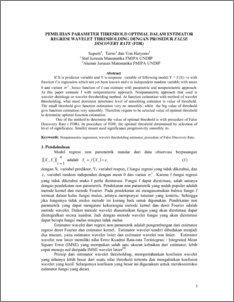# PEMILIHAN PARAMETER THRESHOLD OPTIMAL DALAM ESTIMATOR REGRESI WAVELET THRESHOLDING DENGAN PROSEDUR FALSE DISCOVERY RATE (FDR)

Suparti, Suparti and Tarno, Tarno and Haryono, Yon (2008) PEMILIHAN PARAMETER THRESHOLD OPTIMAL DALAM ESTIMATOR REGRESI WAVELET THRESHOLDING DENGAN PROSEDUR FALSE DISCOVERY RATE (FDR). Media Statistika, 1 (1). pp. 1-9. ISSN 1979-3693Preview
PDF
215Kb

Official URL: http://stat.undip.ac.id

## Abstract

If X is predictor variable and Y is response variable of following model Y = f (X) +e with function f is regression which not yet been known and e is independent random variable with mean 0 and variant , hence function of f can estimate with parametric and nonparametric approach. At this paper estimate f with nonparametric approach. Nonparametric approach that used is wavelet shrinkage or wavelet thresholding method. At function estimation with method of wavelet thresholding, what most dominant determine level of smoothing estimator is value of threshold. The small threshold give function estimation very no smoothly, while the big value of threshold give function estimation very smoothly. Therefore require to be selected value of optimal threshold to determine optimal function estimation. One of the method to determine the value of optimal threshold is with procedure of False Discovery Rate ( FDR). In procedure of FDR, the optimal threshold determined by selection of level of significance. Smaller mount used significance progressively smoothly its . Keywords: Nonparametric regression, wavelet thresholding estimator, procedure of False Discovery Rate.

Item Type: Article Q Science > Q Science (General) Faculty of Science and Mathematics > Department of Statistics 1366 Mr Hasbi Yasin 15 Oct 2009 14:10 15 Oct 2009 14:10

Repository Staff Only: item control page